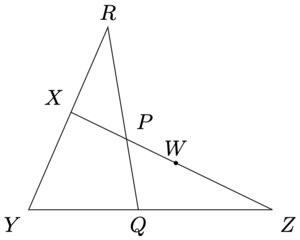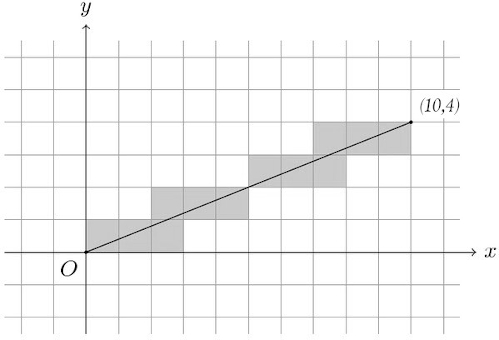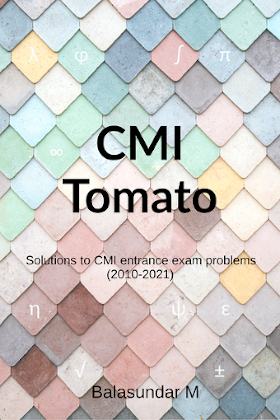# CMI Tomato

This website is for class XI and XII students who wish to pursue B.Sc. at Chennai Mathematical Institute.

Disclaimer. This is an unofficial resource and is not affiliated to CMI.

#### Announcement

• Congrats to everybody to made it to CMI!

## Problems from CMI 2022 paper [Work in progress]

### Part A: Screening part

1. Suppose $$a_0, a_1, a_2, \ldots,$$ is an arithmetic progression such that $$a_0$$ and $$a_1$$ are positive integers. Suppose $$g_0, g_1, a_2, \ldots,$$ is a geometric progression such that $$g_0=a_0$$ and $$g_1=a_1$$. Which of the following are true?
1. $$a_5^2 \geq a_0a_{10}$$
2. The number $$a_0 + a_1 + \cdots + a_{10}$$ is divisible by $$a_5$$.
3. If $$\sum_{i=0}^{\infty} a_i$$ tends to $$\infty$$ then $$\sum_{i=0}^{\infty} g_i$$ tends to $$\infty$$.
4. If $$\sum_{i=0}^{\infty} g_i$$ is finite then $$\sum_{i=0}^{\infty} a_i$$ tends to $$-\infty$$.
Sol.
1. TRUE. $$a_5^2 \geq a_0 \cdot a_{10} \implies 25d^2 \geq 0$$ which is true.
2. TRUE. $$a_0 + 5d \; | \; 11(a + 5d)$$
3. TRUE. AP has a common difference of $$d>0$$, the common ratio of GP is >1.
4. TRUE. gp is finite then common ratio $$<1$$ so $$a_1/a_0 < 1$$ so $$d/a_0$$ is negative so $$d$$ is negative so AP sum goes to -inf.
2. $$A$$ and $$B$$ are probability events such that $$P(A)$$ and $$P(B)$$ is strictly between 0 and 1. We say that the events are mutually exclusive if $$P(A\cap B) = 0$$. We say the events $$A$$ and $$B$$ are exhaustive if $$P(A\cup B)=1$$. Let $$A^c$$ denote the complement of $$A$$.
1. $$A$$ and $$B$$ are mutually exclusive if and only if $$A^c$$ and $$B^c$$ are exhaustive.
2. $$A$$ and $$B$$ are independent if and only if $$A^c$$ and $$B^c$$ are independent.
3. $$A$$ and $$B$$ cannot be simultaneously independent and exhaustive.
4. $$A$$ and $$B$$ cannot be simultaneously mutually exclusive and exhaustive.
Sol.
1. TRUE. $$A \cap B = \phi \iff A^c \cup B^c = S$$
2. TRUE. If $$A$$ and $$B$$ are independent, then $$P(A\cap B) = P(A) \cdot P(B) \implies P(A^c \cap b^c) = P[(A \cup B)^c] = P(A^c) P(B^c)$$.
3. TRUE. Exhaustive means $$P(A \cup B)=1$$. We have $$P(A \cup B) = P(A) + P(B) - P(A \cap B) = 1 - (1-P(A))(1-P(B)) < 1$$. Hence A and B cannot be both exhaustive and independent.
4. FALSE. $$A, B$$ can be both mutually exclusive and exhaustive. Eg $$\{H\}$$ and $$\{T\}$$ are mutually exclusive and exhaustive for a coin toss.
3. Suppose we have a matrix $$A$$ as defined below. Which of the following are true? $A = \begin{bmatrix} 1 & 2 & 3 \\ 10 & 20 & 30 \\ 11 & 22 & k \end{bmatrix}$
1. $$A$$ is not invertible regardless of the value of $$k$$.
2. There exists a unique $$k$$ such that the determinant of $$A$$ is zero.
3. The set of solutions $$\vec{v}=(x,y,z)$$ to $$A\vec{v}=0$$ is either a plane or a line passing through the origin.
4. If $$A\vec{v}=[p\;\; q\;\; r]^{\top}$$ has a solution, then $$q=10p$$.
Sol.
4. TRUE. As the first two rows are multiples of one another, det(A)=0 regardless of k.
5. FALSE. Because A is true.
6. TRUE. $$A\vec{v} = 0$$. If $$k=33$$, then we would get only one equation :- $$x+2y=3$$ means plane passing through origin. If $$k \neq 33$$, we get two distinct equations: $$x+2y+z=0$$ and $$11x+22y+kz=0$$ now combine to give equation of a line via origin.
7. TRUE.
8. Consider the three statements about a function defined on $$[0,1]$$.
(I) $$f$$ is differentiable on $$[0,1]$$.
(II) $$f$$ is continuous on $$[0,1]$$.
(III) $$f$$ attains it maximum and minimum in $$[0,1]$$.
Pick the correct statements.
1. (I) implies (II).
2. (II) implies (III).
3. not (III) implies not (I).
4. No two of the three statements are equivalent to one another.
Sol.
9. TRUE. At every point $f'(x)$ exists so right and left hand continuous.
10. TRUE. It is basically a trivial sorta theorem that every continuous function chives max min in a closed interval.
11. TRUE. if not max min then it is not differentiable.
12. TRUE. I implies II but II doesn't imply I, I implies III but III doesn't imply I, II implies III but III doesn't imply II.
13. Which of these are true?
1. $$a = 1/\ln 3 \implies 3^a = e$$.
2. $$\sin(0.02) < 2\sin(0.01)$$.
3. $$\arctan(0.01) > 0.01$$.
4. $$4 \int_{0}^1 \arctan x \, dx = \pi - \ln 4$$.
Sol.
14. TRUE. $$log_{e}3 = 1/a \implies e^{1/a} = 3 \implies 3^a = e$$
15. TRUE. Can solve this problem by using the on-screen calculator. Alternatively, you may use Taylor expansion.
16. FALSE.
17. TRUE.
18. Which of the options are true about the function $$f$$ as defined below: $f(x) = \frac{1}{|\ln x|} \left( \frac{1}{x} + \cos x \right)$
1. As $$x\rightarrow \infty$$, the sign of $$f$$ changes infinitely many times.
2. $$\lim_{x\rightarrow \infty} f(x)$$ does not exist.
3. $$\lim_{x\rightarrow 1} f(x) = \infty$$.
4. $$\lim_{x\rightarrow 0^+} f(x) = 1$$.
19. Let $$f_0(x) = x$$,$$f_1(x)=x^x$$,$$f_2(x) = x^{x^x}$$, etc. For $$x>0$$ which of the following options are true?
1. $$\lim_{x\rightarrow 0^+} f_1(x) = 1$$
2. $$\lim_{x\rightarrow 0^+} f_2(x) = 1$$
3. $$\int_0^{1} f_3 dx = 1$$
4. $$f_{123}^\prime(1) = 1$$
20. Let $$N=\{1,2,\ldots,9\}$$ and $$L=\{a,b,c\}$$.
1. $$L \cup N$$ is arranged on a line with the letters appearing consecutively (in any order). The number of such arrangements are less than $$10!\times 5$$.
2. More than half of the functions from $$N$$ to $$L$$ have $$b$$ in their range.
3. The number of one-to-one functions from $$L$$ to $$N$$ is less than 512.
4. The number of functions $$N$$ to $$L$$ that do not map consecutive numbers to consecutive letters is greater than 512.
21. Let $$z$$ be a non-real complex number and $$f(z) = z^{222} + \frac{1}{z^{222}}$$. Which of the statements are correct?
1. If $$|z|=1$$, then $$f(z)$$ must be real.
2. If $$z+1/z=1$$, then $$f(z)=2$$.
3. If $$z+1/z$$ is real, then $$|f(z)|\leq 2$$.
4. If $$f(z)$$ is real, then $$f(z)>0$$.
22. Let $$P$$ denote some arrangement of the numbers $${1,2,\ldots,n}$$. A move on $$P$$ consists of exchanging the position of element 1 with the position of another element. For example, if $$P=[3,1,4,2]$$, then by making one move we can get either $$P=[1,3,4,2]$$, $$P=[3,4,1,2]$$ or $$P=[3,2,4,1]$$. $$P$$ is said to be sorted if the numbers are in increasing order. Which of the statements are true?
1. The arrangement $$[9, 1, 2, 3, 4, 5, 6, 7, 8]$$ can be sorted in 8 moves and no fewer.
2. $$[1, 3, 4, 5, 6, 7, 8, 9, 2]$$ can be sorted in 8 moves and no fewer.
3. $$[1, 3, 4, 2, 9, 5, 6, 7, 8]$$ cannot be sorted by any number of moves.
4. There exists an arrangement of 99 numbers, i.e. 1 to 99, that cannot be sorted by any number of moves.

### Part B: Subjective questions

B1. Consider the figure shown below (not drawn to scale). We have the following relation: $\frac{WZ}{YX} = \frac{PW}{XP} = \frac{QZ}{YQ} = k$ Prove that $$XR = XP$$.B2. This question was straight from a previous CMI Tomato's mock test!
(i) Consider a coordinate grid made up of horizontal and vertical lines of the form $$x=k$$ and $$y=k$$, where $$k\in \mathbb{Z}$$. Consider a line segment OA where O is the origin and A is the point $$(10,4)$$. Notice that this line segment passes through the interior of 12 cells. In the figure below, the shaded cells are the ones through which the line passes.In general, how many cells does the line from the origin to $$(m,n)$$ pass through? Assume that $$m$$ and $$n$$ are positive integers.
(ii) Consider all the cells in the square region from $$(0,0)$$ to $$(n,n)$$. What is the maximum number of cells any line can pass through in this region? This line need not pass through any integer points.

B3. Let $$f(x) = 1 + x + x^2 + \cdots + x^n$$. Find the local minima of $$f(x)$$. Find the local maxima of $$f(x)$$. For each local maxima or minima $$(c,f(c))$$, find the integer $$k$$ such that $$k\leq c < k+1$$.

Hint: Use the definition of $$f$$ as well as the closed formula. Treat $$x\geq 0$$ and $$x<0$$ separately.

B4. For a function $$f:\mathbb{R}^+ \rightarrow \mathbb{R}^+$$, define $$A_r$$ and $$B_r$$ as follows: $A_r := \int_{1}^{r} f(x)\, dx \;\;\;\;\; B_r := \int_{r}^{r^2} f(x)\, dx$ Find all continuous functions $$f:\mathbb{R}^+\rightarrow\mathbb{R}^+$$ for which $$A_r=B_r$$ for every positive $$r$$.
Hint: Find an equation relating $$f(x)$$ with $$f(x^2)$$. Then consider the function $$xf(x)$$.

B5. A pair of distinct real numbers $$(r, s)$$ is considered to be a good pair if $r^{3}+s^{2}=s^{3}+r^{2}$ (i) Find a good pair $$(a, l)$$ such that $$l$$ takes the maximum value possible.
Find a good pair $$(s,b)$$ such that $$s$$ takes the minimum value possible.
For any good pair $$(c, d)$$, other than the ones found above, show that there exists another real number $$e$$ such that $$(c, e)$$ and $$(d, e)$$ are both good pairs.
(ii) Prove that there are infinitely many rational good pairs.

Hints: Consider the function $$f=x^3-x^2$$.
(i) For a good pair $$(r,s)$$, can you write $$s$$ in terms of $$r$$?
(ii) You can use the fact that there are infinitely many right-angle triangles with integer sides that are not similar to each other.

B6. Let $$p$$ denote a prime number in this question.
(i) Show that $$x^2+x-1$$ has at most two roots $$\pmod{p}$$. In other words show that the cardinality of the set $$S$$ (defined below) is at most two. $S = \{ n\in \mathbb{N} | \;\;\; 1\leq n \leq p, \;\; s.t. \;\;\; p|n^2+n-1 \}$ Also, find all the primes $$p$$ for which the cardinality of $$S$$ is one.
(ii) Find all $$n\leq 121$$ such that $$n^2+n-1$$ is divisible by $$121$$.
(iii) What can we say about the roots of $$x^2+x-1$$ $$\pmod{p^2}$$?

#### What’s on this site?

• In-depth solutions to previous CMI entrance exam questions. This includes questions from 2010 and 2011 for which official solutions are not given.

• Topic-wise classification of all problems ordered by increasing difficulty. This helps you to specialize in a topic.

## Browse previous years' problems

Hover on the cell to see the problem. Click on it to navigate to the solution.
Algebra
Geometry
Number theory
Calculus
Combinatorics
Probability
Complex numbers
Trigonometry

## CMI entrance exam cutoff

The cutoff has been around 40% for the objective section and 40-50% overall. The objective section is used for screening.

CMI does not publish the cutoff marks but they have responded to individual requests in the past. The scores shown below were shared by former students who were privy to this data.

### 2021 cutoff marks

In 2021, CMI used an unconventional criteria for admission. The cut-off for Part A was 17/40 for everyone. More weightage was given to Part B marks. In the general category with scholarship, students were admitted if they had >=61 marks in total or if they had 39 or more marks in Part B. So, a student who got 17 marks in Part A and 39 marks in Part B was still admitted with scholarship. But a student with 22 marks in Part A and 38 marks in Part B was NOT admitted in that category.

TypeGeneralReserved
With scholarship61/100 or 39/60 in Part B57/100 or 35/60 in Part B
Without scholarship56/100 or 30/60 in Part B50/100 or 28/60 in Part B

There was no waiting list.

### 2020 cutoff marks

The cutoff marks for the year 2020 are given below. The marks are out of 94 instead of 100 since one question had a mistake.

TypeGeneralReserved
With scholarship54/9444/94
Without scholarship48/9440/94
Waiting list45/9438/94

The cutoff marks for the previous years are given below.

YearObjective (40 marks)OverallVetted by
20161462/124Sayantani Bhattacharya
20171560/125Abhiroop Sanyal
20181550/125-
2019∼1652/100Ankush Agarwal
2020∼1648/94CMI Office
20211756/100CMI Office

## Preparation tips and interview experiences (pre-2016)

• 2015 Interview experience by Sanjay M who posted this on AoPS. [pdf]
• Why I joined CMI by Vipul Naik who joined CMI despite getting JEE AIR-154.
• How to prepare for CMI by Sai Krishna who joined CMI in 2013. [pdf]
• CMI entrance exam preparation experience by Ankita Sarkar who joined CMI in 2015.

Starting from 2016, admissions are based only on the written test and there is no interview.When universities in the United States are looking to recruit mathematics students from India, one of the first places they look for applications is from CMI, if not the first place. Universities that are able to get CMI students are always very proud.

Manjul Bhargava

Source

Acceptance offers are sent to around 90 candidates and about half of them enroll. This is the reason why the number varies from year to year. Approximate numbers are given below.

Year# Test-takers# Offers# Students enrolled
201540007040
201640009044
201740009045
201850009548
2019550010061

## PaperbackThe book contains the problems and solutions from the years 2010-2021.

If you have a more elegant solution to any problem than the one presented, feel free to email him at mbalasundar08 [at] gmail.com.